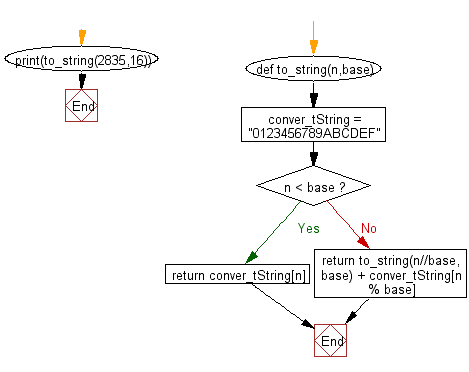﻿ Python Data Structures and Algorithms - Recursion: Convert an integer to a string in any base - w3resource# Python Data Structures and Algorithms - Recursion: Convert an integer to a string in any base

## Python Recursion: Exercise-2 with Solution

Write a Python program to converting an integer to a string in any base.

Sample Solution:

Python Code:

``````def to_string(n,base):
conver_tString = "0123456789ABCDEF"
if n < base:
return conver_tString[n]
else:

print(to_string(2835,16))
```
```

Sample Output:

```B13
```

Flowchart:## Visualize Python code execution:

The following tool visualize what the computer is doing step-by-step as it executes the said program:

Python Code Editor:

What is the difficulty level of this exercise?

Test your Programming skills with w3resource's quiz.

﻿

## Python: Tips of the Day

Decapitalizes the first letter of a string:

Example:

```def tips_decapitalize(s, upper_rest=False):
return s[:1].lower() + (s[1:].upper() if upper_rest else s[1:])
print(tips_decapitalize('PythonTips'))
print(tips_decapitalize('PythonTips', True))
```

Output:

```pythonTips
pYTHONTIPS
```